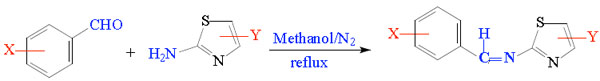Eq. (1) Describes schematic diagram for the preparation of Schiff bases (No. 1-15),: X, 1 = -H, 2 = 2-OH, 3 = 4-NO2, 4 = 4-Br, 5 = 3-OH, 6= 2-NO2, 7 = 3-Me (compounds from: 1-7, Y = H) 8, Y = 4-CH3 (X = H), 9, Y = 4-Me (X = 2-OH), 10, Y = 4-Me (X = 4-NO2), 11, Y = 4-Me, (X = 4-Br), 12, Y = 5-Me, (X = H), 13, Y = 5-Me, (X = 2-OH), 14, Y = 5-Me, (X = 4-NO2), 15, Y = 5-Me, (X = 4-Br).